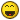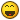Geometry question regarding right triangles

Recommended Posts

This is a question that has been perplexing me for a number of years. Unfortunately, I don't have the experience with geometry to answer it myself, so I am hoping someone here can help.

Note: No this is not homework help - It's the basis for another idea that's been percolating around in my brain, which I may add to the end of the thread, assuming this turns out the way I expect.

Let us suppose we have a right triangle, ABC, with sides AB and BC, and hypotenuse AC.

If we draw one line that bisects and is perpendicular to AB and another that bisects and is perpendicular to BC, will the intersection of those lines fall exactly on AC?

Edited by Greg H.
Share on other sites

Yes it will. In fact they will fall on the mid point of AC. If you call the mid point of AC "O" then lines OA OB and OC are radii of a circle. You can work from there to prove your statement.

Edited by Joatmon
Share on other sites

Yes it will. In fact they will fall on the mid point of AC. If you call the mid point of AC "O" then lines OA OB and OC are radii of a circle. You can work from there to prove your statement.

That's what I was hoping, actually. Following that line of reasons, the area of the quadrilateral bounded by AB, BC, and the two bisecting lines should be exactly half the area of the triangle ABC.

If we assign the values of a and b to AB and BC, then:

AreaABC = 1/2ab

So the area of the quadrilateral would be

Areaquad = 1/2a * 1/2b = 1/4ab

Is this true for any triangle, or is it another of those special cases for right triangles?

Share on other sites

I think the quadrilateral you are trying to describe is bounded by part of AB part of BC and the two bisecting lines. This is, in fact a rectangle because all angles are 90 degrees.

It can be proved that this rectangle has half the area of the right triangle ABC. (you can use "mirror image" congruent triangles using the mid point of AC).

Since angle ABC is 90 degrees you are correct in saying the area of ABC is 1/2(a*b)

Therefore you are correct in saying the area of the rectangle is 1/4(a*b)

Out of interest if you made a complete circle that goes through A B and C you could complete a rectangle using A B C and the other semicircle. If you extend your bisecting lines to the other sides of this rectangle you will see that your "quadrilateral" is a quarter of this new rectangle. This new rectangle has an area ab, so once again you get 1/4ab as the answer you want!

Be wary - all this concerns right triangles.

Share on other sites

Thanks Joat. That more or less confirms the back of the napkins drawings I have been puzzling at for a few years. I was never more than a passing student of geometry (my brain, it seems, is not a fan of Euclid), so I never had the knowledge needed to prove what seemed logical to me.

I appreciate the confirmation.

Share on other sites

Greg the point that you are forming - ie where the two perpendicular bisectors cross is known as the circumcentre (in fact the third will also cross at it). A triangles circumcentre is the centre of the unique circle which has all three points on it. This applies to all triangles; for a right-angled triangle (as you have spotted) the circumcentre is located at the midpoint of the hypotenuse, an acute triangle will have the circumcentre inside, and an obtuse triangle (that has an angle over 90degrees) will have a circumcentre outside the triangle. I don't know much about the areas of the sectors created by those lines - but I am sure we could work it out if you need.

So what was the other thing?

Share on other sites

Actually, the other thing was the area of the quadrilateral being exactly equal to one-half the area of the triangle. Joat already answered it for me.

I like math, so I tend to nibble at ideas that seem interesting to me. This one came to me one afternoon when I was doodling during a conference call. I think I vaguely remember the term circumcentre from my geometry classes, but they were more years ago than I care to count. I may have to re-familiarize myself with the concept.

As a further exercise, supposing I once again have a right triangle, ABC, whose sides are of known lengths a & b.

Given the pythagorean theorem, it is possible that I can compute the area and circumference of the circumcircle (I went and read a bit on them) in the following manner:

$A = \pi r^2$

$C = \pi d$

Since we know that $a^2 + b^2 = c^2$ and for a right triangle, we know that the radius of the circumcircle equals exactly $\frac{1}{2} c$, we can say

$A = \pi\left(\frac{1}{2}\sqrt{a^2 + b^2} \right)^2$

and

$C = \pi\left(\sqrt{a^2 + b^2} \right)$

Or am I talking out of my butt here?

Share on other sites

I would go along with thatShare on other sites

• 1 month later...

This is a question that has been perplexing me for a number of years. Unfortunately, I don't have the experience with geometry to answer it myself, so I am hoping someone here can help.

Note: No this is not homework help - It's the basis for another idea that's been percolating around in my brain, which I may add to the end of the thread, assuming this turns out the way I expect.

Let us suppose we have a right triangle, ABC, with sides AB and BC, and hypotenuse AC.

If we draw one line that bisects and is perpendicular to AB and another that bisects and is perpendicular to BC, will the intersection of those lines fall exactly on AC?

Yes it will. In a right triangle, the circumcenter always lies on the hypotenuse.

Create an account

Register a new account